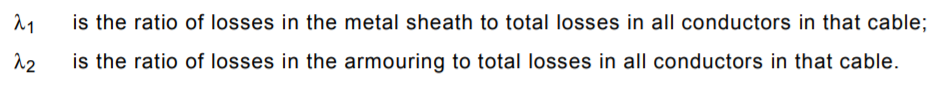This solution article relates to using Cable HV Software.

Background:

Circulating currents occur in metallic cable components such as the sheath, concentric neutral or armour layers and only when the bonding arrangement is solidly bonded.

According to the IEC Standard 60287-1-1 calculation methods there are two main loss factors defined:These lambda factors are a ratio to the conductor losses.  Therefore if we know these factors and we know the conductor loss then we can convert these factors into a loss or even a current.

Step 1.  First thing we do is examine the Losses of the cable.

In this example below the conductor loss is 46.806738 W/m which is caused by the flow of the conductor current and the conductor resistance (I^2.R losses).  Note this is a single core cable.

To calculate the concentric neutral loss we multiply the circulating current loss factor of 0.145587 with the conductor loss above to get the Concentric neutral loss of 6.81445 W/m.

You can try this yourself:

0.145587 * 46.806738 = 6.81445 W/m.

Step 2.  Calculate the circulating current from the losses.

The losses in the concentric neutral layer (Pn) are generated no differently to conductor losses; they too are based on I^2R losses.  In other words:

Pn(losses) = I^2 * Rn

Where Rn is the resistance of the concentric neutral which is also calculated and given in the results below.

Transposing the above equation to make current the subject:

In = sqrt(Pn/Rn) = sqrt(6.81445/0.000311553)

Therefore, the circulating current in the concentric neutral is equal to 147.8936 A.

When there are multiple earthed metallic layers in the cable then the circulating currents for all these layers are calculated in the same manner and summed to give the total circulating current value.

Take care when dealing with multicore cables with individual screens.